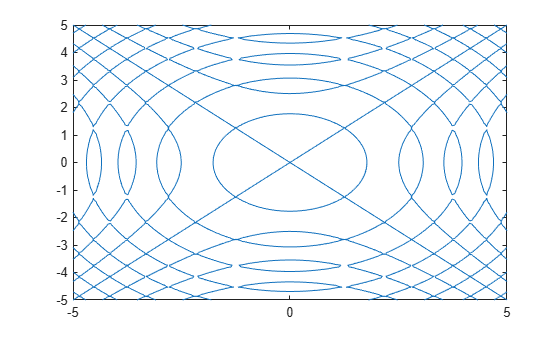eq

Define symbolic equation

Description

example

A == B defines a symbolic equation. Use the equation as input to functions such as solve, assume, fcontour, and subs.

eq(A,B) is equivalent to A == B.

Examples

collapse all

Solve this trigonometric equation. Define the equation by using the == operator.

syms x
eqn = sin(x) == cos(x);
solve(eqn,x)
ans =
pi/4

Plot the equation $\mathrm{sin}\left({x}^{2}\right)=\mathrm{sin}\left({y}^{2}\right)$ by using fimplicit. Define the equation by using the == operator.

syms x y
eqn = sin(x^2) == sin(y^2);
fimplicit(eqn)Test the equality of two symbolic expressions by using isAlways.

syms x
eqn = x+1 == x+1;
isAlways(eqn)
ans =
logical
1
eqn = sin(x)/cos(x) == tan(x);
isAlways(eqn)
ans =
logical
1

Check the equality of two symbolic matrices by using isAlways.

A = sym(hilb(3));
B = sym([1 1/2 5; 1/2 2 1/4; 1/3 1/8 1/5]);
isAlways(A == B)
ans =
3×3 logical array
1     1     0
1     0     1
1     0     1

Compare a matrix and a scalar. The == operator expands the scalar into a matrix of the same dimensions as the input matrix.

A = sym(hilb(3));
B = sym(1/2);
isAlways(A == B)
ans =
3×3 logical array
0     1     0
1     0     0
0     0     0

Input Arguments

collapse all

Input, specified as a number, vector, matrix, or array, or a symbolic number, scalar variable, matrix variable (since R2021a), array, function, or expression.

Input, specified as a number, vector, matrix, or array, or a symbolic number, scalar variable, matrix variable (since R2021a), array, function, or expression.

Tips

• Calling == or eq for nonsymbolic A and B invokes the MATLAB® eq function. This function returns a logical array with elements set to logical 1 (true) where A and B are equal; otherwise, it returns logical 0 (false).

• If both A and B are arrays, then they must have the same dimensions. A == B returns an array of equations A(i,j,...) == B(i,j,...).